Win up to 100% scholarship on Aakash BYJU'S JEE/NEET courses with ABNAT Win up to 100% scholarship on Aakash BYJU'S JEE/NEET courses with ABNAT

# JEE Main 2022 July 29 – Shift 2 Physics Question Paper with Solutions

The JEE Main 2022 July 29 – Shift 2 Physics Question Paper with Solutions is provided on this page. Solving the JEE Main 2022 question papers helps students to boost their preparation. JEE Main 2022 answer keys contain the exact solutions to the JEE questions in a step-by-step method. Students can easily understand and learn these JEE Main 2022 question papers with solutions and thus improve their knowledge in each subject. Revise the JEE Main 2022 July 29 – Shift 2 Physics Question Paper with Solutions given below.

## JEE Main 2022 29th July Shift 2 Physics Question Paper and Solutions

SECTION – A

Multiple Choice Questions: This section contains 20 multiple choice questions. Each question has 4 choices (1), (2), (3) and (4), out of which ONLY ONE is correct.

1. Two identical metallic spheres A and B when placed at certain distance in air repel each other with a force of F. Another identical uncharged sphere C is first placed in contact with A and then in contact with B and finally placed at midpoint between spheres A and B. The force experienced by sphere C will be

(A) 3F/2

(B) 3F/4

(C) F

(D) 2F

Sol. When two identical sphere come in contact with each other, the total charge on them is equally distribute.$$\begin{array}{l}\frac{kQ^2}{d^2}=F\end{array}$$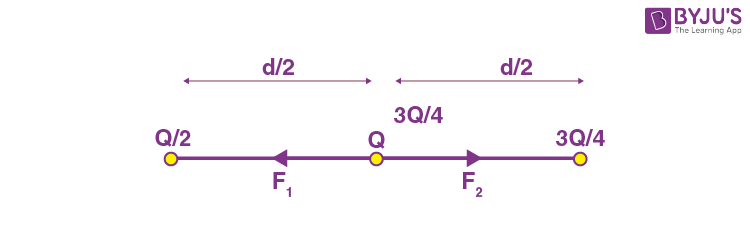$$\begin{array}{l}F’=\frac{k9Q^2}{16\times \frac{d^2}{4}}-\frac{k3Q^2}{8 \times \frac{d^2}{4}} \end{array}$$
$$\begin{array}{l}=\frac{9kQ^2}{4d^2}-\frac{3kQ^2}{2d^2}\end{array}$$
$$\begin{array}{l}=\frac{kQ^2}{d^2}[\frac{9}{4}-\frac{3}{2}]\end{array}$$
$$\begin{array}{l}=\frac{6}{8}F=\frac{3}{4}F\end{array}$$

2. Match List I with List II.

List I List II
A. Torque I. Nms–1
B. Stress II. Jkg–1
C. Latent Heat III. Nm
D. Power IV Nm–2

Choose the correct answer from the options given below:

(A) A-III, B-II, C-I, D-IV

(B) A-III, B-IV, C-II, D-I

(C) A-IV, B-I, C-III, D-II

(D) A-II, B-III, C-I, D-IV

Sol. Torque → Nm

Stress → N/m2

Latent heat → J/kg

Power → N m/s

A-III, B-IV, C-II, D-I

3. Two identical thin metal plates has charge q1 and q2 respectively such that q1 > q2. The plates were brought close to each other to form a parallel plate capacitor of capacitance C. The potential difference between them is

$$\begin{array}{l}(\text{A})\ \frac{(q_1+q_2)}{C}\end{array}$$
$$\begin{array}{l}(\text{B})\ \frac{(q_1-q_2)}{C}\end{array}$$
$$\begin{array}{l}(\text{C})\ \frac{(q_1-q_2)}{2C}\end{array}$$
$$\begin{array}{l}(\text{D})\ \frac{2(q_1-q_2)}{C}\end{array}$$

Sol.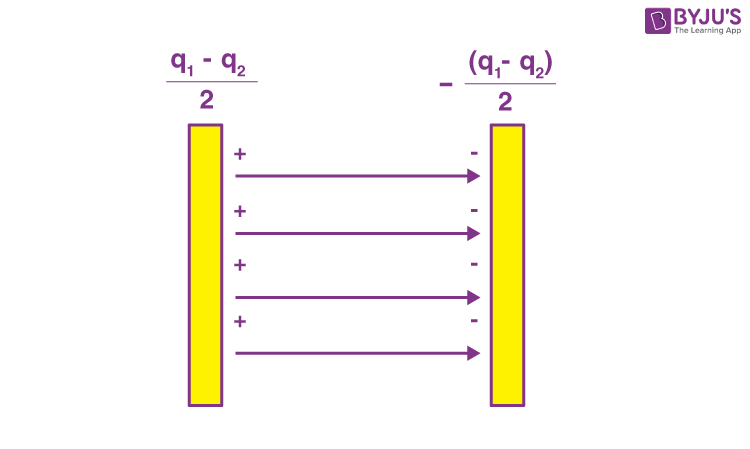$$\begin{array}{l}E=\frac{q_1-q_2}{2\varepsilon_0A}\end{array}$$
$$\begin{array}{l}v=\frac{(q_1-q_2)d}{2\varepsilon_0A}\end{array}$$
$$\begin{array}{l}=\frac{q_1-q_2}{2C}\end{array}$$

4. Given below are two statements: one is labelled as Assertion A and the other is labelled as Reason R.

Assertion A: Alloys such as constantan and manganin are used in making standard resistance coils.

Reason R: Constantan and manganin have very small value of temperature coefficient of resistance.

In the light of the above statements, choose the correct answer from the options given below.

(A) Both A and R are true and R is the correct explanation of A.

(B) Both A and R are true but R is NOT the correct explanation of A.

(C) A is true but R is false.

(D) A is false but R is true.

Sol. Since they have low temperature coefficient of resistance, their resistance remains almost constant.

5. A 1 m long wire is broken into two unequal parts X and Y. The X part of the wire is stretched into another wire W. Length of W is twice the length of X and the resistance of W is twice that of Y. Find the ratio of length of X and Y.

(A) 1:4

(B) 1:2

(C) 4:1

(D) 2:1

Sol.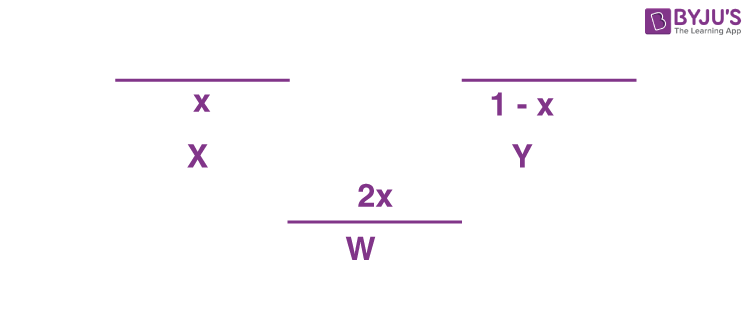Rw = 2Ry

$$\begin{array}{l}\rho\frac{2x}{\frac{A}{2}}=\frac{2\rho(1-x)}{A}\end{array}$$

4x = 2(1 – x)

$$\begin{array}{l}\frac{x}{1-x}=\frac{1}{2}\end{array}$$

6. A wire X of length 50 cm carrying a current of 2 A is placed parallel to a long wire Y of length 5 m. The wire Y carries a current of 3 A. The distance between two wires is 5 cm and currents flow in the same direction. The force acting on the wire Y is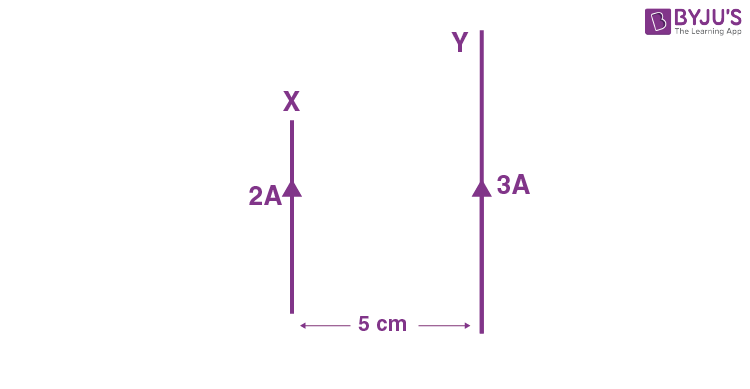(A) 1.2 × 10–5 N directed towards wire X

(B) 1.2 × 10–4 N directed away from wire X

(C) 1.2 × 10–4 N directed towards wire X

(D) 2.4 × 10–5 N directed towards wire X

Sol. FXY = FYX = F

$$\begin{array}{l}F=\frac{\mu_0I_2}{2\pi r}I_1(l)\end{array}$$
$$\begin{array}{l}=\frac{4\pi \times 10^{-7} \times 3 \times 2 \times [50 \times 10^{-2}]}{2\pi (5\times 10^{-2})}\end{array}$$

= 1.2 × 10–5 N

7. A juggler throws balls vertically upwards with same initial velocity in air. When the first ball reaches its highest position, he throws the next ball. Assuming the juggler throws n balls per second, the maximum height the balls can reach is

(A) g/2n

(B) g/n

(C) 2gn

(D) g/2n2

Sol.

$$\begin{array}{l}t=\frac{u}{g}=\frac{1}{n}\end{array}$$
$$\begin{array}{l}u=\frac{g}{n}\end{array}$$
$$\begin{array}{l}H_{\text{max}}=\frac{u^2}{2g}=\frac{9}{2n^2}\end{array}$$

8. A circuit element X when connected to an a.c. supply of peak voltage 100 V gives a peak current of 5 A which is in phase with the voltage. A second element Y when connected to the same a.c. supply also gives the same value of peak current which lags behind the voltage by π/2. If X and Y are connected in series to the same supply, what will be the rms value of the current in ampere?

$$\begin{array}{l}(\text{A})\ \frac{10}{\sqrt{2}}\end{array}$$
$$\begin{array}{l}(\text{B})\ \frac{5}{\sqrt{2}}\end{array}$$
$$\begin{array}{l}(\text{C})\ 5\sqrt{2}\end{array}$$
$$\begin{array}{l}(\text{D})\ \frac{5}{2}\end{array}$$

Sol.

$$\begin{array}{l}R=\frac{100}{5}=20\ \Omega\end{array}$$
$$\begin{array}{l}X_L=\frac{100}{5}=20\ \Omega\end{array}$$

When in series

$$\begin{array}{l}z=\sqrt{20^2 + 20^2}=20\sqrt{2}\ \Omega\end{array}$$
$$\begin{array}{l}i = \frac{100}{z}=\frac{100}{20\sqrt{2}}=\frac{5}{\sqrt{2}}\end{array}$$
$$\begin{array}{l}i_{\text{rms}}= \frac{1}{\sqrt{2}}i\end{array}$$
$$\begin{array}{l}=\frac{5}{2}\end{array}$$

9. An unpolarised light beam of intensity 2I0 is passed through a polaroid P and then through another polaroid Q which is oriented in such a way that its passing axis makes an angle of 30° relative to that of P. The intensity of the emergent light is

$$\begin{array}{l}(\text{A})\ \frac{I_0}{4}\end{array}$$
$$\begin{array}{l}(\text{B})\ \frac{I_0}{2}\end{array}$$
$$\begin{array}{l}(\text{C})\ \frac{3I_0}{4}\end{array}$$
$$\begin{array}{l}(\text{D})\ \frac{3I_0}{2}\end{array}$$

Sol.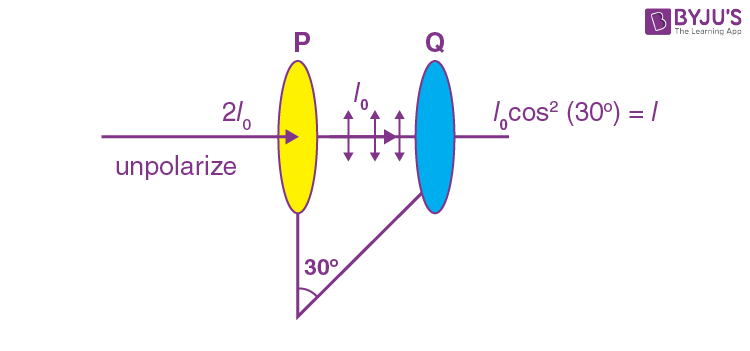$$\begin{array}{l}I = I_0 \times \frac{3}{4}\end{array}$$

10. An α particle and a proton are accelerated from rest through the same potential difference. The ratio of linear momenta acquired by above two particles will be:

$$\begin{array}{l}(\text{A})\ \sqrt{2}:1\end{array}$$
$$\begin{array}{l}(\text{B})\ 2\sqrt{2}:1\end{array}$$
$$\begin{array}{l}(\text{C})\ 4\sqrt{2}:1\end{array}$$

(D) 8 : 1

Sol.

$$\begin{array}{l}\frac{p_\alpha}{p_p}=\frac{\sqrt{2(4m)(2eV)}}{\sqrt{2(m)(eV)}}\end{array}$$
$$\begin{array}{l}=\frac{\sqrt{16}}{\sqrt{2}}\end{array}$$
$$\begin{array}{l}=\frac{4}{\sqrt{2}} = \frac{2\sqrt{2}}{1}\end{array}$$

(A) Volume of the nucleus is directly proportional to the mass number.

(B) Volume of the nucleus is independent of mass number.

(C) Density of the nucleus is directly proportional to the mass number.

(D) Density of the nucleus is directly proportional to the cube root of the mass number.

(E) Density of the nucleus is independent of the mass number.

Choose the correct option from the following options

(A) (A) and (D) only

(B) (A) and (E) only

(C) (B) and (E) only

(D) (A) and (C) only

Sol.

$$\begin{array}{l}R=R_0A^{1/3}\end{array}$$
$$\begin{array}{l}\Rightarrow V = \frac{4}{3}\pi R^3 = \frac{4}{3}\pi R_0^3A\end{array}$$
$$\begin{array}{l}\Rightarrow \rho = \frac{M}{V}\propto \frac{A}{A}\propto A^0\end{array}$$

12. An object of mass 1 kg is taken to a height from the surface of earth which is equal to three times the radius of earth. The gain in potential energy of the object will be

[If, g = 10 ms–2 and radius of earth = 6400 km]

(A) 48 MJ

(B) 24 MJ

(C) 36 MJ

(D) 12 MJ

Sol.

$$\begin{array}{l}\Delta U = U_f – U_i\end{array}$$
$$\begin{array}{l}=-\frac{GMm}{4R}+\frac{GMm}{R}\end{array}$$
$$\begin{array}{l}=\frac{3GMm}{4R}=\frac{3}{4}mgR\end{array}$$

= 48 MJ

13. A ball is released from a height h. If t1 and t2 be the time required to complete first half and second half of the distance respectively. Then, choose the correct relation between t1 and t2.

$$\begin{array}{l}(\text{A})\ t_1 = (\sqrt{2})t_2\end{array}$$
$$\begin{array}{l}(\text{B})\ t_1 = (\sqrt{2} – 1)t_2\end{array}$$
$$\begin{array}{l}(\text{C})\ t_2 = (\sqrt{2} + 1)t_1\end{array}$$
$$\begin{array}{l}(\text{D})\ t_2 = (\sqrt{2} – 1)t_1\end{array}$$

Sol.

$$\begin{array}{l}t_1=\sqrt{\frac{2\cdot\frac{H}{2}}{g}} = \sqrt{\frac{H}{g}}\end{array}$$
$$\begin{array}{l}\text{And } t_2 = \sqrt{\frac{2H}{g}} -t_1\end{array}$$
$$\begin{array}{l}\Rightarrow t_2 = \sqrt{\frac{2H}{g}}-\sqrt{\frac{H}{g}}=\sqrt{\frac{H}{g}}\{\sqrt{2}-1\}\end{array}$$

14. Two bodies of masses m1 = 5 kg and m2 = 3 kg are connected by a light string going over a smooth light pulley on a smooth inclined plane as shown in the figure. The system is at rest. The force exerted by the inclined plane on the body of mass m1 will be
[Take g = 10 ms–2]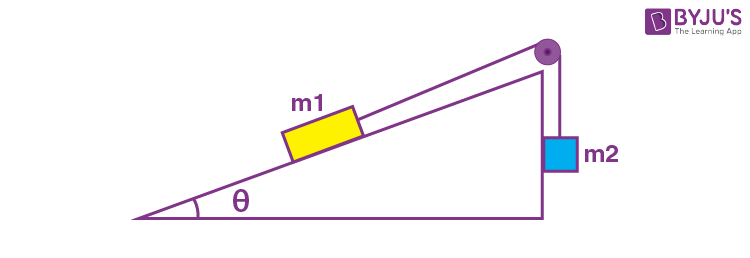(A) 30 N

(B) 40 N

(C) 50 N

(D) 60 N

Sol. m2g = m1gsinθ …(i)

N = m1gcosθ …(ii)

$$\begin{array}{l}\Rightarrow \frac{N}{m_2g}=\cot \theta\end{array}$$
$$\begin{array}{l}\Rightarrow N = 3\times 10 \times \cot \theta = 3 \times 10 \times \frac{4}{3}\ \ \ \left( \because \sin \theta =\frac{3}5{} \right)\end{array}$$

N = 40 Newtons

15. If momentum of a body is increased by 20%, then its kinetic energy increases by

(A) 36%

(B) 40%

(C) 44%

(D) 48%

Sol.

$$\begin{array}{l}K = \frac{p^2}{2m}\end{array}$$
$$\begin{array}{l}K’ = \frac{(1.2p)^2}{2m}\end{array}$$
$$\begin{array}{l}\Rightarrow \frac{K’-K}{K}=(1.2)^2 -1 = 0.44\end{array}$$

⇒ 44% increase

16. The torque of a force

$$\begin{array}{l}5\hat{i}+3\hat{j}-7\hat{k}\end{array}$$
about the origin is τ. If the force acts on a particle whose position vector is
$$\begin{array}{l}2\hat{i}+2\hat{j}+\hat{k},\end{array}$$
then the value of τ will be

$$\begin{array}{l}(\text{A})\ 11\hat{i}+19\hat{j}-4\hat{k}\end{array}$$
$$\begin{array}{l}(\text{B})\ -11\hat{i}+9\hat{j}-16\hat{k}\end{array}$$
$$\begin{array}{l}(\text{C})\ -17\hat{i}+19\hat{j}-4\hat{k}\end{array}$$
$$\begin{array}{l}(\text{C})\ 17\hat{i}+9\hat{j}+16\hat{k}\end{array}$$

Sol.

$$\begin{array}{l}\vec{\tau} = \begin{vmatrix}\hat{i} & \hat{j} & \hat{k} \\2 & 2 & 1 \\5 & 3 & -7 \\\end{vmatrix}\end{array}$$
$$\begin{array}{l}=\hat{i}(-14-3)+\hat{j}(5+14)+\hat{k}(6-10)\end{array}$$
$$\begin{array}{l}=-17\hat{i}+19\hat{j}-4\hat{k}\end{array}$$

17. A thermodynamic system is taken from an original state D to an intermediate state E by the linear process shown in the figure. Its volume is then reduced to the original volume from E to F by an isobaric process. The total work done by the gas from D to E to F will be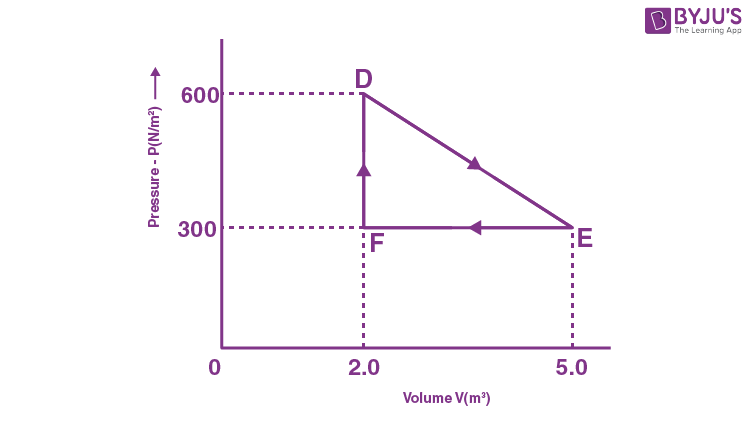$$\begin{array}{l}\left(A\right) -450~\text{J}\\ \left(B\right) 450~\text{J}\\ \left(C\right) 900~\text{J}\\ \left(D\right) 1350~\text{J}\end{array}$$

Sol.

$$\begin{array}{l}W=\frac{1}{2}\times (5-2)\times(600 – 300) \text{ J}\end{array}$$
$$\begin{array}{l}=\frac{1}{2}\times 3 \times 300= 450 \text{J} \end{array}$$

18. The vertical component of the earth’s magnetic field is 6 × 10–5 T at any place where the angle of dip is 37°. The earth’s resultant magnetic field at that place will be (Given tan 37° = ¾)

$$\begin{array}{l}\left(A\right) 8 \times 10^{–5}~\text{T}\\ \left(B\right)6 \times 10^{–5}~\text{T}\\ \left(C\right)5 \times 10^{–4}~\text{T}\\ \left(D\right)1 \times 10^{–4}~\text{T}\end{array}$$

Sol.

$$\begin{array}{l}B_v = B_0sin\theta\end{array}$$
$$\begin{array}{l}B_0 = \frac{B_v}{\sin \theta} = \frac{6 \times 10^{-5}}{\sin 37^{\circ}}\end{array}$$
$$\begin{array}{l}=\frac{6 \times 10^{-5}}{3}\times 5\end{array}$$
$$\begin{array}{l} = 1 \times 10^{–4}~\text{T} \end{array}$$

19. The root mean square speed of smoke particles of mass 5 × 10-17 in their Brownian motion in air at NTP is approximately. [Given k = 1.38 × 10-23 JK-1]

$$\begin{array}{l}\left(A\right) 60 ~\text{mm s}^{–1}\\ \left(B\right) 12~ \text{mm s}^{–1}\\ \left(C\right)15~ \text{mm s}^{–1}\\ \left(D\right) 36~ \text{mm s}^{–1}\end{array}$$

Sol.

$$\begin{array}{l}\text{At NTP}, T = 298~ \text{K}\\\Rightarrow v_{\text{rms}}= \sqrt{\frac{3RT}{M}}\end{array}$$
$$\begin{array}{l}=\sqrt{\frac{3kN_A \times 298}{5 \times 10^{-17}\times N_A}}\end{array}$$
$$\begin{array}{l}\simeq 15 \text{ mm/s}\end{array}$$

20. Light enters from air into a given medium at an angle of 45° with interface of the air-medium surface. After refraction, the light ray is deviated through an angle of 15° from its original direction. The refractive index of the medium is

(A) 1.732

(B) 1.333

(C) 1.414

(D) 2.732

Sol.

$$\begin{array}{l}1 \times sin 45^\circ = \mu \times sin 30^\circ\end{array}$$
$$\begin{array}{l}\Rightarrow \mu = \frac{1 }{\sqrt{2}}\times \frac{2}{1}\end{array}$$
$$\begin{array}{l}\mu = \sqrt{2}\\= 1.414 \end{array}$$

SECTION – B

Numerical Value Type Questions: This section contains 10 questions. In Section B, attempt any five questions out of 10. The answer to each question is a NUMERICAL VALUE. For each question, enter the correct numerical value (in decimal notation, truncated/rounded-off to the second decimal place; e.g. 06.25, 07.00, –00.33, –00.30, 30.27, –27.30) using the mouse and the on-screen virtual numeric keypad in the place designated to enter the answer.

1. A tube of length 50 cm is filled completely with an incompressible liquid of mass 250 g and closed at both ends. The tube is then rotated in horizontal plane about one of its ends with a uniform angular velocity

$$\begin{array}{l}x\sqrt{F} \text{ rad s}^{-1} .\end{array}$$
If F be the force exerted by the liquid at the other end then the value of x will be______.

Sol.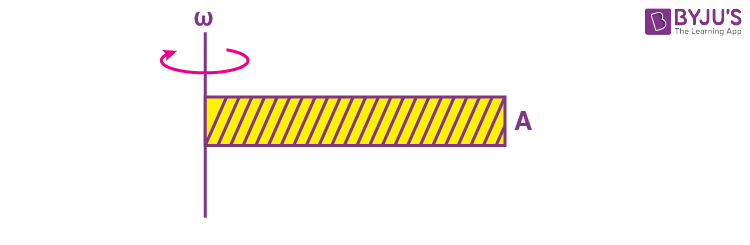$$\begin{array}{l}\text{Applying } F_c = \frac{m\omega ^2 l}{2}\end{array}$$
$$\begin{array}{l} \frac{m\omega ^2 l}{2} = F\end{array}$$
$$\begin{array}{l}\omega = \sqrt{\frac{2F}{\frac{1}{2}\times \frac{1}{4}}}=\sqrt{16 F} = 4\sqrt{F}\end{array}$$

2. Nearly 10% of the power of a 110 W light bulb is converted to visible radiation. The change in average intensities of visible radiation, at a distance of 1 m from the bulb to a distance of 5 m is a × 10–2 W.m2. The value of ‘a‘ will be _____.

Sol.

$$\begin{array}{l}P_{radiation} = 0.1 \times 110 = 11~\text{W}\end{array}$$
$$\begin{array}{l}\Delta I_{\text{radiation}_1} = I_{\text{radiation}_1} – I_{\text{radiation}_2}\end{array}$$
$$\begin{array}{l}=11\left( \frac{1}{4\pi} – \frac{1}{4\pi \times 25}\right)=\frac{11 \times 24}{4\pi \times 25}\\= 84 \times 10^{–2}~ \text{W/m}^2\end{array}$$

3. A metal wire of length 0.5 m and cross-sectional area 10–4 m2 has breaking stress 5 × 108 Nm–2. A block of 10 kg is attached at one end of the string and is rotating in a horizontal circle. The maximum linear velocity of block will be _____ ms–1.

Sol.

$$\begin{array}{l} A = 10^{–4} \text{m}^2\\l = \frac{1}{2} \text{ m}\\\sigma = 5 × 10^8\end{array}$$
$$\begin{array}{l}\frac{mv^2}{lA}=5 \times 10^8\end{array}$$
$$\begin{array}{l}v=\sqrt{\frac{5\times 10^8 \times \frac{1}{2}\times 10^{-4}}{10}}=5 \times 10 = 50 \text{ m/s}\end{array}$$

4. The velocity of a small ball of mass 0.3 g and density 8 g/cc when dropped in a container filled with glycerine becomes constant after some time. If the density of glycerine is 1.3 g/cc, then the value of viscous force acting on the ball will be x × 10–4 N. The value of x is _______. [use g = 10 m/s2]

Sol.

$$\begin{array}{l} F_v = 6\pi \eta rv_T\\ F_v + F_B = mg\\ \Rightarrow Fv = mg – F_B\end{array}$$
$$\begin{array}{l}=10 \times (8 – 1.3 )\times \frac{0.3}{8}\times 10^{-3}\\= 2.5125 \times 10^{–3}\ \text{N} \end{array}$$
$$\begin{array}{l}\simeq 25 \times 10^{-4} \text{ N}\end{array}$$

5. A modulating signal 2sin (6.28 × 106) t is added to the carrier signal 4sin(12.56 × 109) t for amplitude modulation. The combined signal is passed through a non-linear square law device. The output is then passed through a band pass filter. The bandwidth of the output signal of band pass filter will be ______MHz.

Sol.

$$\begin{array}{l} W_C = 12.56 \times 10^9\\ W_m = 6.25 \times 10^6\end{array}$$

After amplitude modulation

Bandwidth frequency

$$\begin{array}{l}=\frac{2W_m}{2\pi}=\frac{2 \times 6.28}{2\pi}\times 10^6=2 \text{ MHz}\end{array}$$

6. The speed of a transverse wave passing through a string of length 50 cm and mass 10 g is 60 ms–1. The area of cross-section of the wire is 2.0 mm2 and its Young’s modulus is 1.2 × 1011 Nm–2. The extension of the wire over its natural length due to its tension will be x × 10–5 m. The value of x is _____.

$$\begin{array}{l}v=\sqrt{\frac{T}{\mu}}\end{array}$$
$$\begin{array}{l}\text{So}\ T = 60^2 \times \frac{10 \times 10^{-3}}{0.5}=72 \text{ N}\end{array}$$
$$\begin{array}{l}\Delta \ell = \frac{T\ell}{YA}= \frac{72 \times 0.5}{1.2 \times 10^{-11}\times 2 \times 10^{-6}} = 15 \times 10^{-5} \text{ m}\end{array}$$

7. The metallic bob of simple pendulum has the relative density 5. The time period of this pendulum is 10 s. If the metallic bob is immersed in water, then the new time period becomes

$$\begin{array}{l}5\sqrt{x} \text{ s}\end{array}$$
. The value of x will be _____.

$$\begin{array}{l}T = 2\pi \sqrt{\frac{\ell}{g}}=10\end{array}$$
$$\begin{array}{l}T’ = 2\pi \sqrt{\frac{ℓ\ell}{g\left(1- \frac{1}{\rho}\right)}}\end{array}$$
$$\begin{array}{l}=2\pi \sqrt{\frac{\ell}{g}\times \frac{5}{4}}=10\sqrt{\frac{5}{4}}=5\sqrt{5}\end{array}$$

8. A 8 V Zener diode along with a series resistance R is connected across a 20 V supply (as shown in the figure). If the maximum Zener current is 25 mA, then the minimum value of R will be ____ Ω.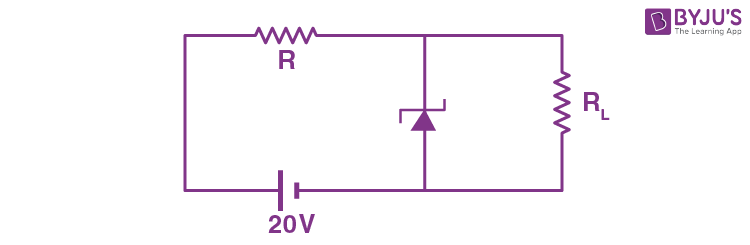R will be minimum when RL is infinitely large, so

$$\begin{array}{l}R_{\text{Zener}} = \frac{8}{25\times 10^{-3}}=320 \ \Omega\end{array}$$
$$\begin{array}{l}\text{So } \frac{R}{R_{\text{Zener}}}=\frac{12}{8}\end{array}$$
$$\begin{array}{l}R=\frac{12}{8}\times 320 = 480\ \Omega\end{array}$$

9. Two radioactive materials A and B have decay constants 25λ and 16λ respectively. If initially they have the same number of nuclei, then the ratio of the number of nuclei of B to that of A will be ‘e’ after a time 1/(aλ). The value of a is _____.

$$\begin{array}{l}N_A = N_0e^{-25\lambda t}\end{array}$$
$$\begin{array}{l}N_B = N_0e^{-16\lambda t}\end{array}$$
$$\begin{array}{l}\frac{N_B}{N_A}=e=e^{9\lambda t}\end{array}$$
$$\begin{array}{l}t = \frac{1}{9\lambda}\end{array}$$

10. A capacitor of capacitance 500 μF is charged completely using a dc supply of 100 V. It is now connected to an inductor of inductance 50 mH to form an LC circuit. The maximum current in the LC circuit will be ______A.

q0 = CV

= 500 × 100 × 10-6 C

= 5 × 10-2 C

For imax,

$$\begin{array}{l}\frac{1}{2}Li_m^2 = \frac{1}{2}\frac{q_0^2}{C}\end{array}$$
$$\begin{array}{l}50 \times 10^{-3}\times i_m^2 = \frac{(5\times 10^{-2})^2}{500 \times 10^{-6}}\end{array}$$
$$\begin{array}{l}\Rightarrow i_m = \frac{5 \times 10^{-2}}{5 \times 10^{-3}}=10 \text{ A}\end{array}$$

### Download PDF of JEE Main 2022 July 29 Shift 2 Physics Paper & Solutions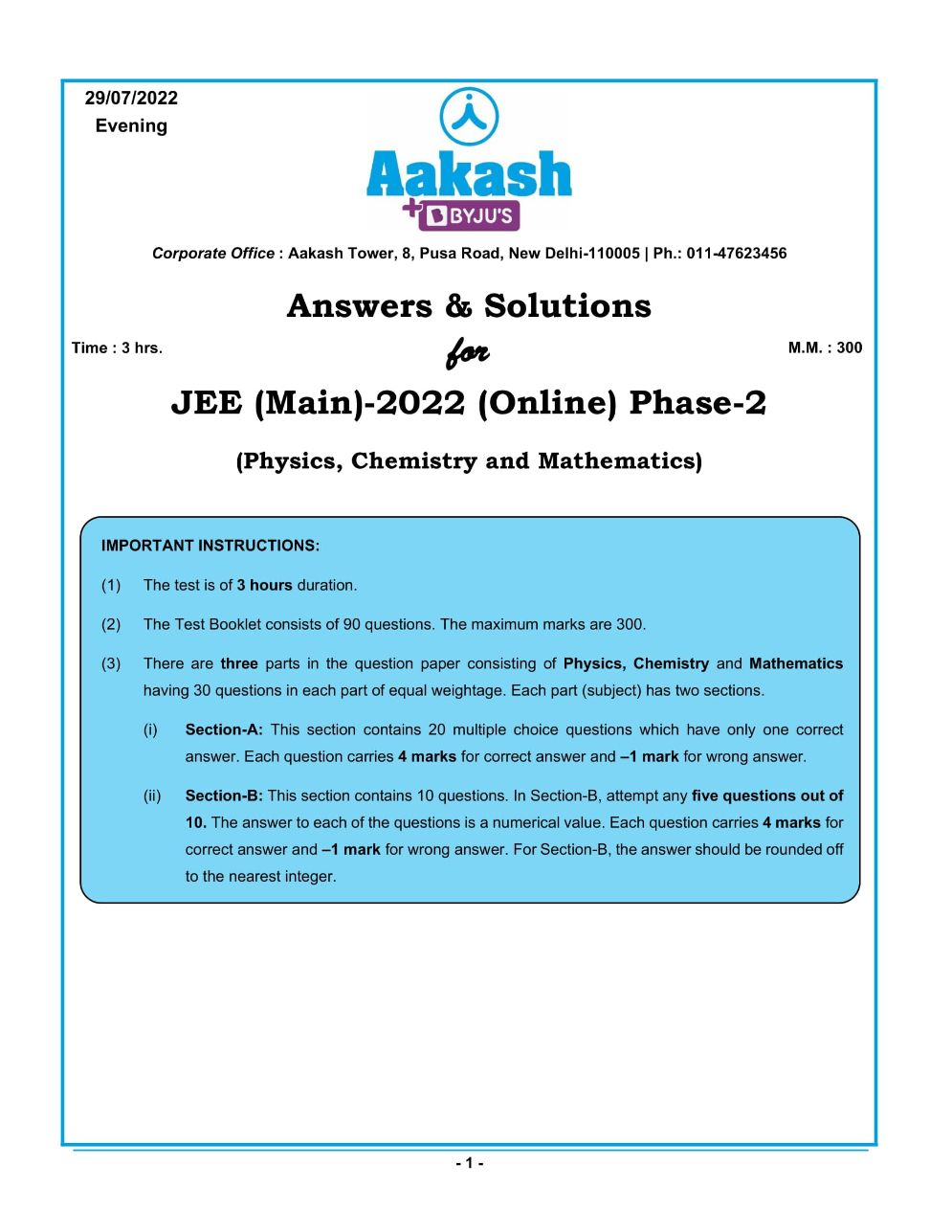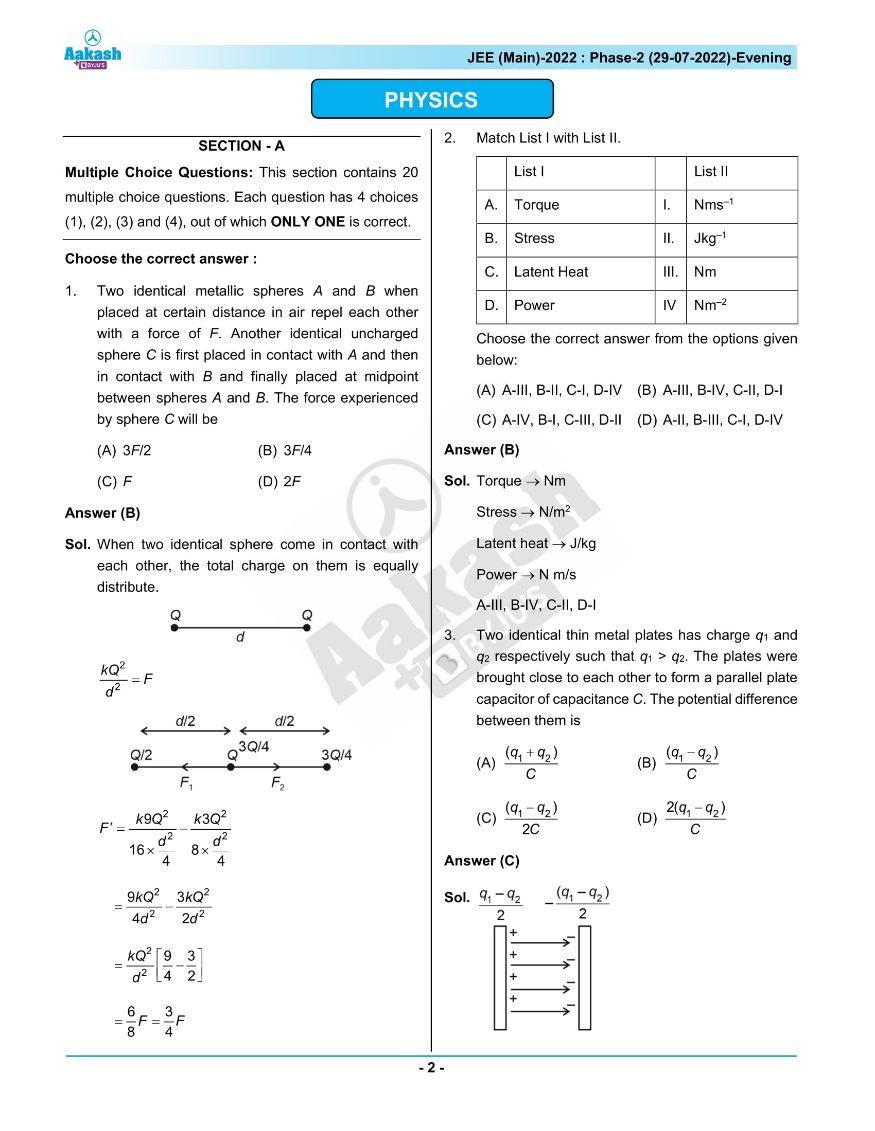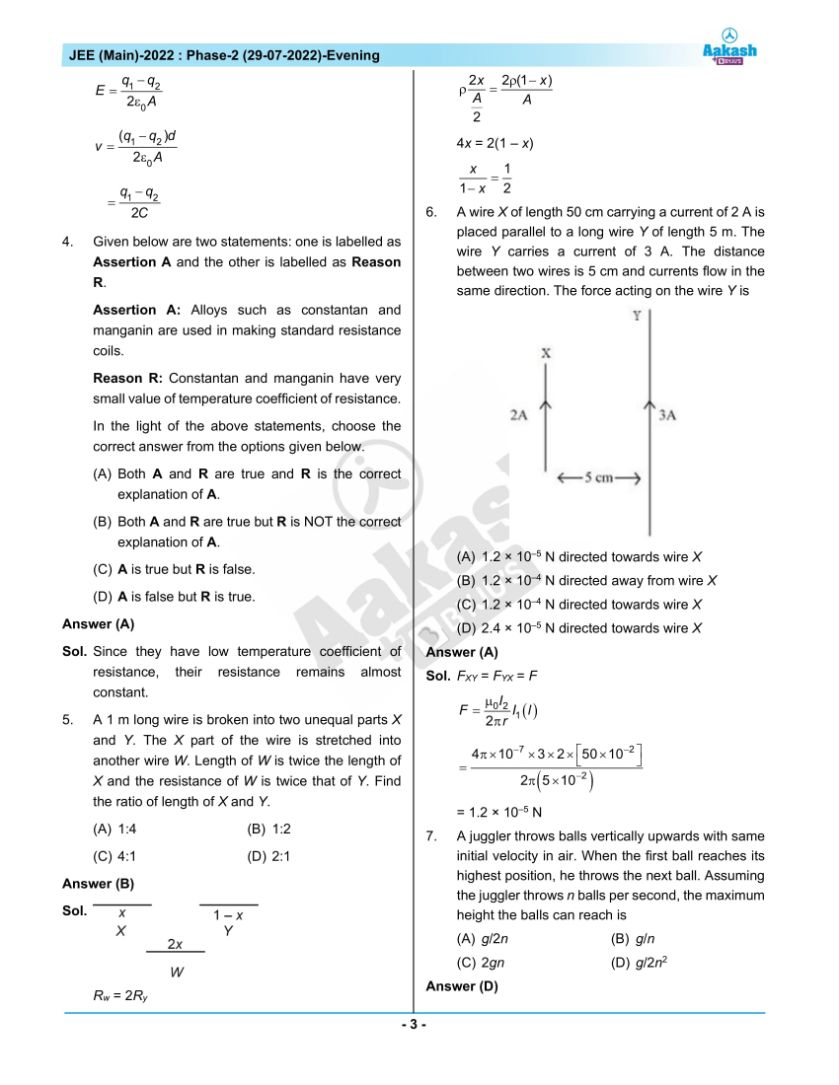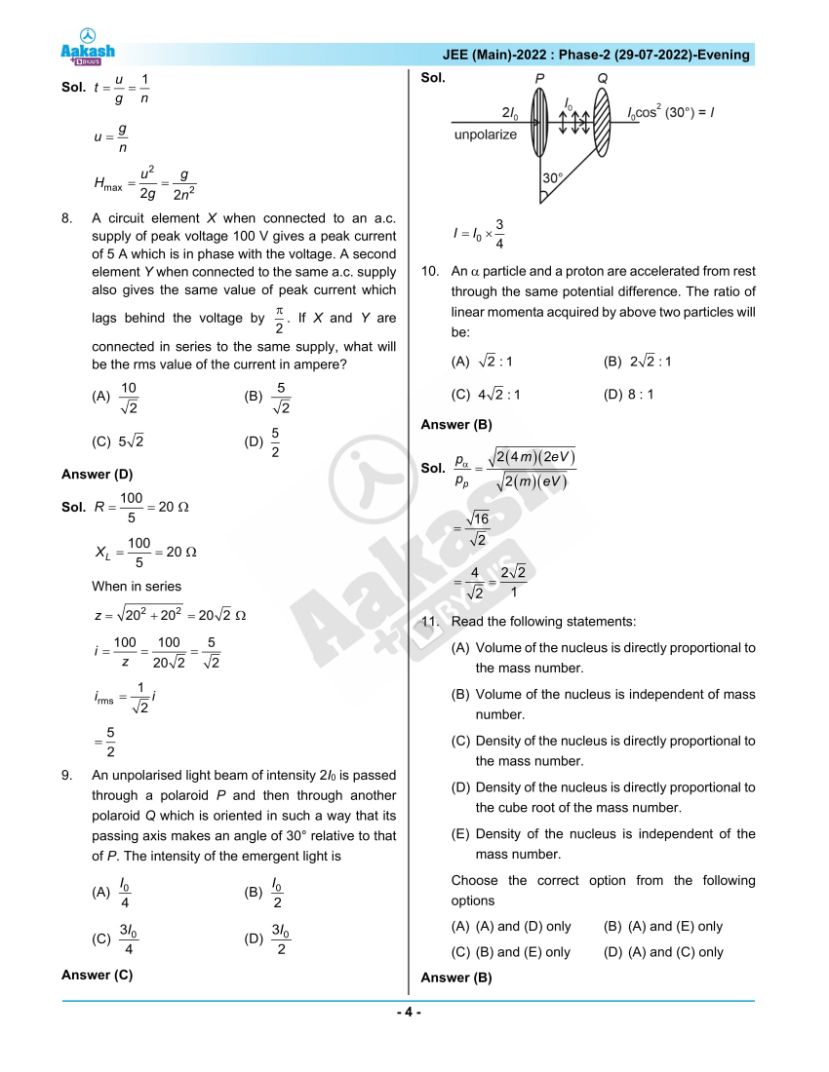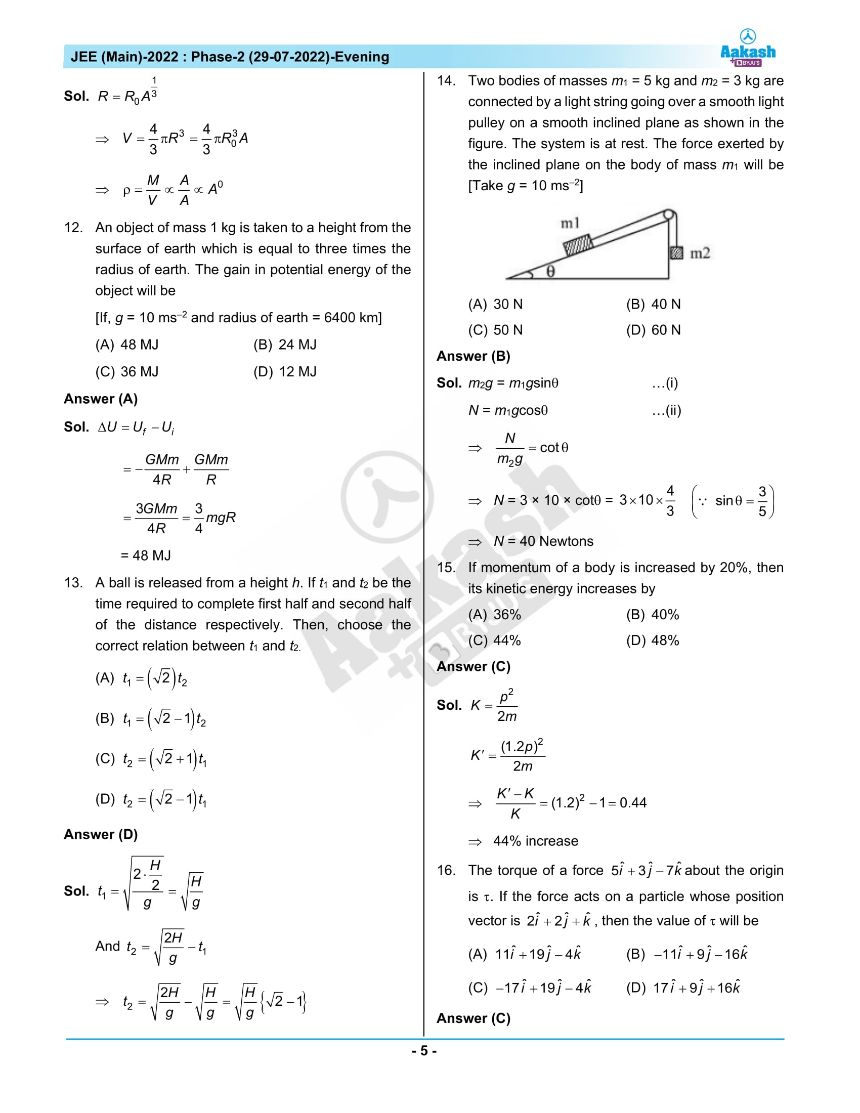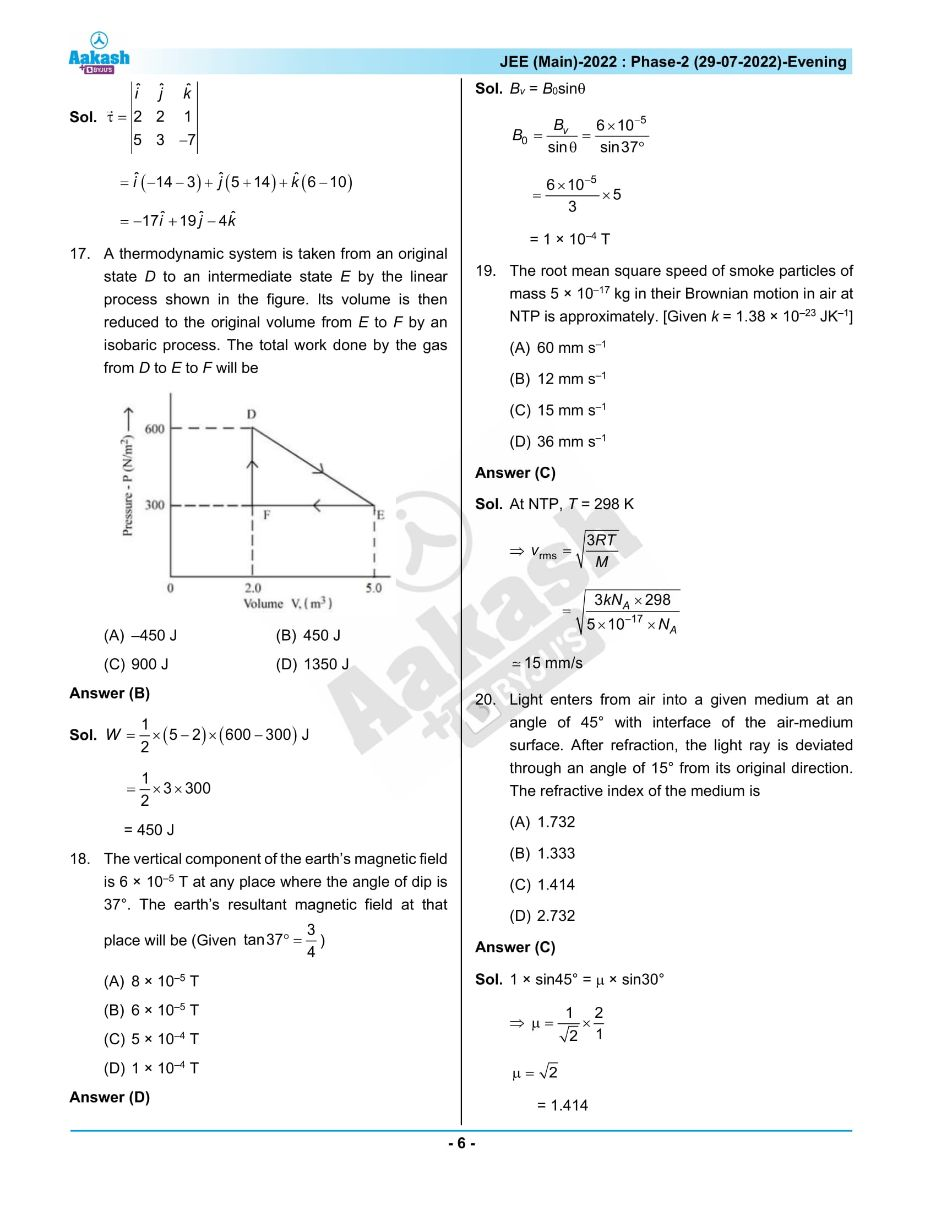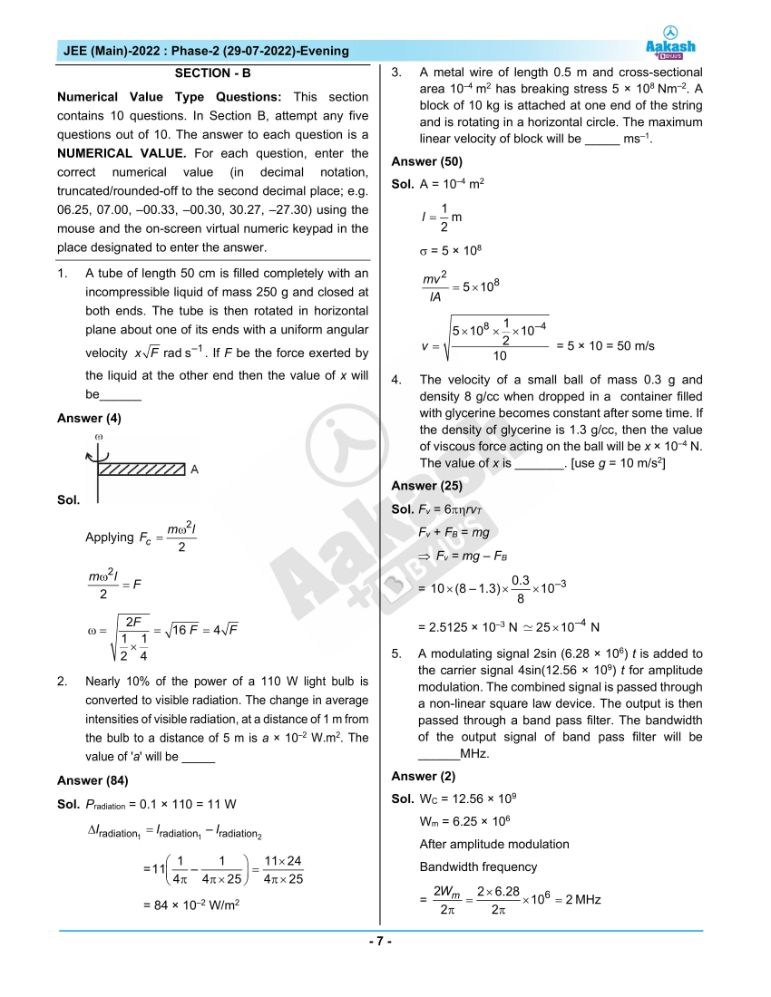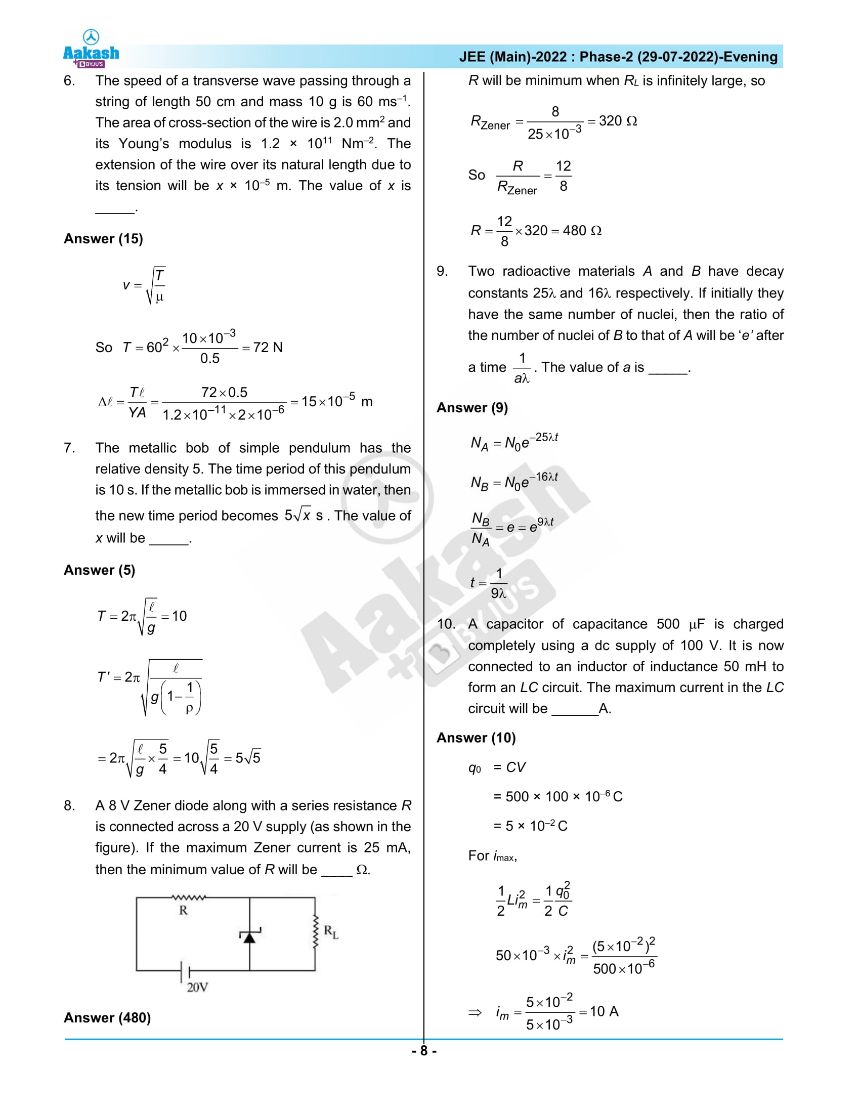## JEE Main 2022 July 29th Shift 2 Paper Analysis